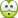# Triognometric problem with vectors (how to solve)

• pennywise1234
In summary, the conversation discusses the use of graphical and trigonometric solutions to calculate the total muscle force vector from three quadriceps muscles with specific forces and angles. The conversation also touches on the concepts of coplanarity, directions, and the correct way to draw vector components.

## Homework Statement

Use the graphical and trigonometic solutions to calculate the total muscle force vector from thefollowing three quadriceps muscles i) rectus femoris pulling 75 N at 5°laterally from the vertical, ii)vastus lateralis pulling 50 N at 30°laterally from the vertical, and iii) vastus medialis pulling 45 N at 40° medially from the vertical.

## Homework Equations

i used basic trig to solve for each vector

## The Attempt at a Solution

I got all vertical and horizontal components for each vector for the 75N at 5 degrees i go 75 horizontal and no vertical
for the 50N at 30 degrees i got 25 vertical and no horizontal

for 45N at 45 degrees i got 28.9 vertical and 34.5 horizontal

i added all the vertical and horizontal square rooted to get the resultant and was of by 10, so where or what did i do wrong ?

pennywise1234 said:
calculate the total muscle force vector from thefollowing three quadriceps muscles i) rectus femoris pulling 75 N at 5°laterally from the vertical, ii)vastus lateralis pulling 50 N at 30°laterally from the vertical, and iii) vastus medialis pulling 45 N at 40° medially from the vertical
A knowledge of physiology tells you that these all act coplanar, does it? I assume they all act on the same side of the vertical, too?

What exactly does medially from the vertical mean here?
pennywise1234 said:
for the 75N at 5 degrees i go 75 horizontal and no vertical
Wouldn't it require a 0° angle to give a zero component along one axis?

well medially in anatomical terms mean closest to the body. but i drew all vectors the on the same quadrant. i thought with 75N and 5 degrees would leave no room to draw a vertical component so i assumed no vertical and the just a horizontal (which would be 75N)

pennywise1234 said:
well medially in anatomical terms mean closest to the body. but i drew all vectors the on the same quadrant. i thought with 75N and 5 degrees would leave no room to draw a vertical component so i assumed no vertical and the just a horizontal (which would be 75N)
Hmm, that sounds a bit rough-and-ready.Where you approximate vertically to 0N it would actually be 6.54N using trigonometry.

ok thank you, i have to ask when if the vertical or horizontal components point to the left of down do count them as negative when doing your math?

That's usually the convention yes. Assuming up and right are positive.

so if i am drawing a vector that is 175N at 190 degree, the 175N is negative?

pennywise1234 said:
so if i am drawing a vector that is 175N at 190 degree, the 175N is negative?

No, the 175 is not negative because you have a direction, which is the 190 degrees. The magnitude of the vector, which is the 175 in this case, is negative when you are comparing it with another direction that you have assigned as positive. For example, if you broke up the vector into its components going along the horizontal and vertical axis then those components could be assigned negative values if you established that right and up were going to be positive directions intially.

pennywise1234 said:
so if i am drawing a vector that is 175N at 190 degree, the 175N is negative?
No. You first break it into its vertical and horizontal components, then decide whether either of those components lies in a negative direction.

i usually draw the vertical and horizontal components away from the origin, is that a correct way to do it?

pennywise1234 said:
i usually draw the vertical and horizontal components away from the origin, is that a correct way to do it?
Vectors can be translated, so translating them to the origin is correct.

•pennywise1234